Courses

# Hots Questions- Quadrilaterals Class 9 Notes | EduRev

## Class 9 : Hots Questions- Quadrilaterals Class 9 Notes | EduRev

The document Hots Questions- Quadrilaterals Class 9 Notes | EduRev is a part of the Class 9 Course Mathematics (Maths) Class 9.
All you need of Class 9 at this link: Class 9

Q.1. If the bisectors of the angles of a quadrilateral enclose a rectangle, then show that it is a parallelogram.
Sol.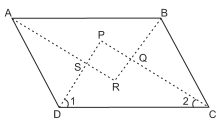Angle bisectors of the quadrilateral ABCD enclose a rectangle PQRS.
∴ ∠P = 90o
⇒ In Δ PCD,  ∠1+∠2 = 90o
But, ∠1 and ∠2 are 1/2∠D and 1/2 ∠C respectively.
⇒ ∠D +∠C = 1800 [∵ 2∠1 + 2∠2 = 180o]
⇒ ∠ D and ∠ C form a pair of co-interior supplementary angles AD || BC
Similarly, AB || DC ⇒ ABCD is a ||gm.

Q.2. L, M, N, K are midpoints of sides BC, CD, DA and AB respectively of a square ABCD.
Prove that DL, DK, BM and BN enclose a rhombus.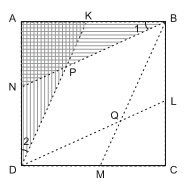Sol.
BK = DM [halves of equal sides]
∴ BM || DK. Similarly, BN || DL
Also, ∆ ABN ≌ ∆ ADK   [SAS congruency]
⇒ ∠ 1 = ∠ 2
Also, ∆PND ≌ ∆PKB     [ASA congruency]
⇒ PB = PD
⇒ DQBP is a rhombus.

Q.3. The sides AD and BC of a quadrilateral are produced as shown in the given figure. Prove that x = ((a+b)/2).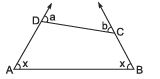Sol.
We have ∠a + ∠ADC = 180°            [linear pair]
Similarly, ∠b + ∠BCD = 180°
But     x + x + ∠ADC + ∠BCD = 360°                   ...(2)
From (1) and (2)
x + x + ∠ADC + ∠BCD = a + b + ∠ADC + ∠BCD
⇒ x + x = a + b
⇒ 2x = a + b
⇒ x = ((a+b)/2)

Q.4. In the adjoining figure, ABCD is a ||gm. Find the angles A, B, C and D.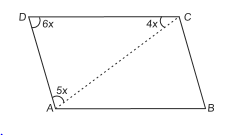Sol.
In ∆ACD, 4x + 5x + 6x = 180°
⇒ 15x = 180°
⇒ x = (180o/15) = 12°
∴ ∠D = 6 × 12° = 72°
⇒ B = 72°                 [∵ opposite angles of ||gm are equal]
∵ ∠ A + ∠ D = 180°                 [cointerior angles]
∴ ∠A = 180° – ∠D = 180° – 72° = 180°
⇒ ∠ C = 108°

Q.5. PQRS is a ||gm. PS is produced to M so that SM = SR and MR produced meet PQ produced at N. Prove that QN = QR.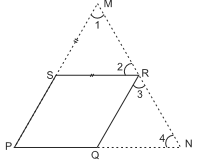Sol.
In ∆SMR, SM = SR
⇒ ∠ 1 = ∠ 2                 [Angles opposite to equal sides are equal]
∠1 = ∠3
[∵QR || PM, corresponding angles are equal]
Similarly, ∠2 = ∠4      [corresponding angles]
⇒ ∠ 3 = ∠ 4
⇒ in ∆ QRN, QN = QR

Offer running on EduRev: Apply code STAYHOME200 to get INR 200 off on our premium plan EduRev Infinity!

## Mathematics (Maths) Class 9

47 videos|324 docs|82 tests

,

,

,

,

,

,

,

,

,

,

,

,

,

,

,

,

,

,

,

,

,

;